Principal Component Analysis PCA Markku HautaKasari Jussi Parkkinen

• Slides: 23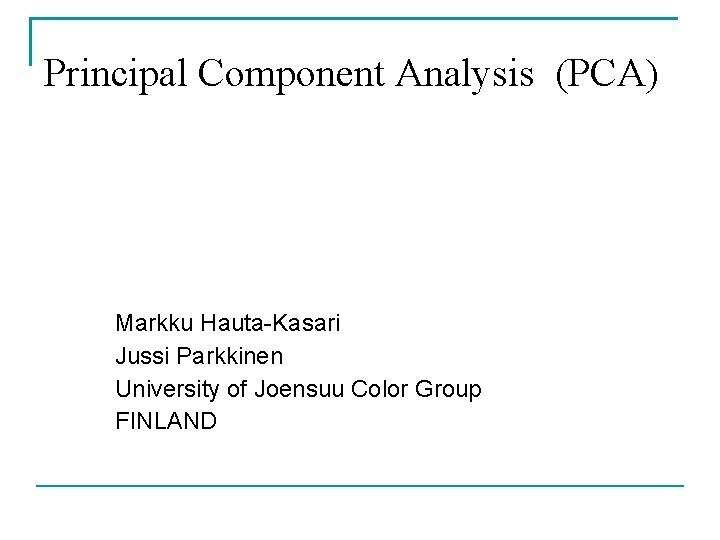Principal Component Analysis (PCA) Markku Hauta-Kasari Jussi Parkkinen University of Joensuu Color Group FINLANDAlgorithm 1. 2. 3. 4. Calculate correlation matrix for the data set Calculate eigenvalues and eigenvectors for the correlation matrix Select eigenvectors corresponding to the largest eigenvalues as a new basis for the data set Calculate principal components between eigenvectors and the data set Applications: compression, pattern recognition etc. Frequently used for spectral image dataAn example on calculating the PCA Example on PCA for the data set of 2 dimensional vectors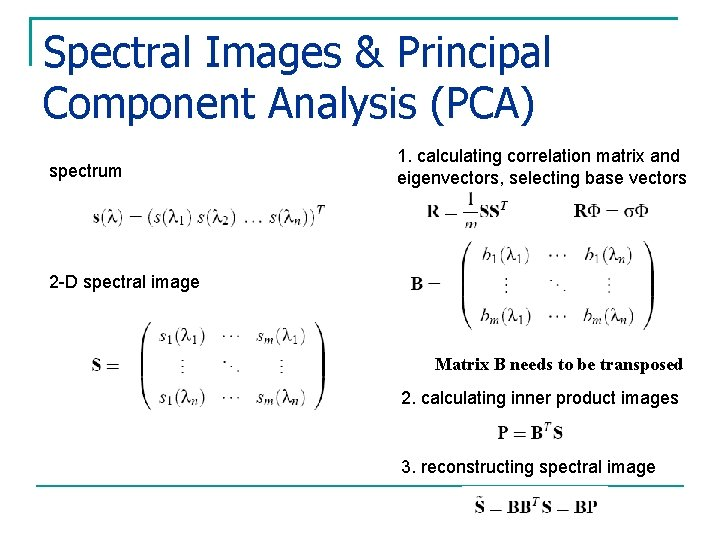Spectral Images & Principal Component Analysis (PCA) spectrum 1. calculating correlation matrix and eigenvectors, selecting base vectors 2 -D spectral image Matrix B needs to be transposed 2. calculating inner product images 3. reconstructing spectral image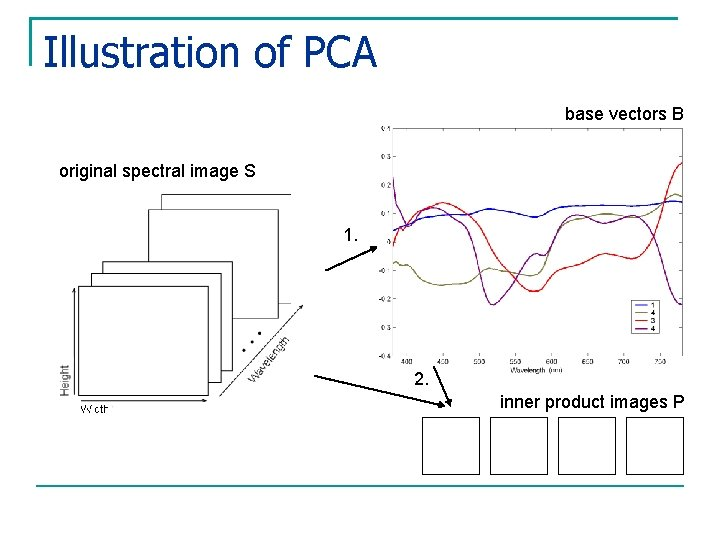Illustration of PCA base vectors B original spectral image S 1. 2. inner product images P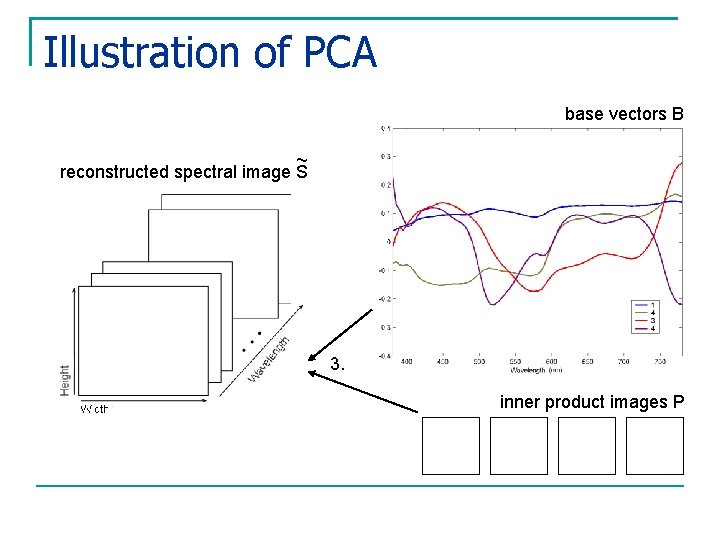Illustration of PCA base vectors B ~ reconstructed spectral image S 3. inner product images P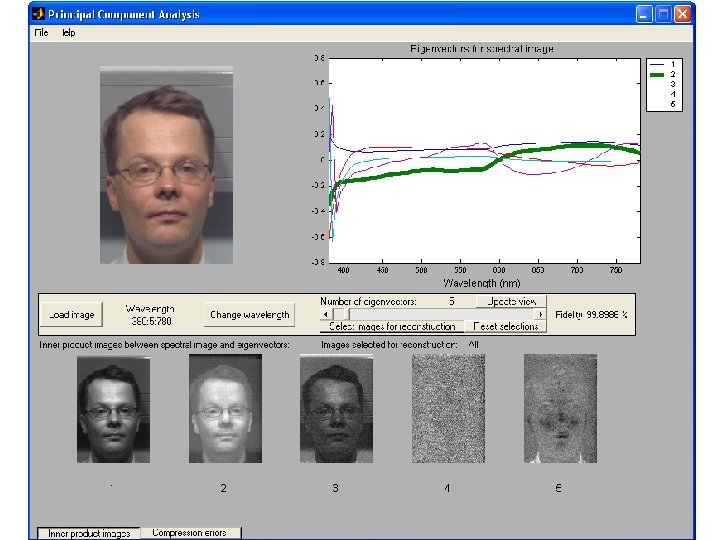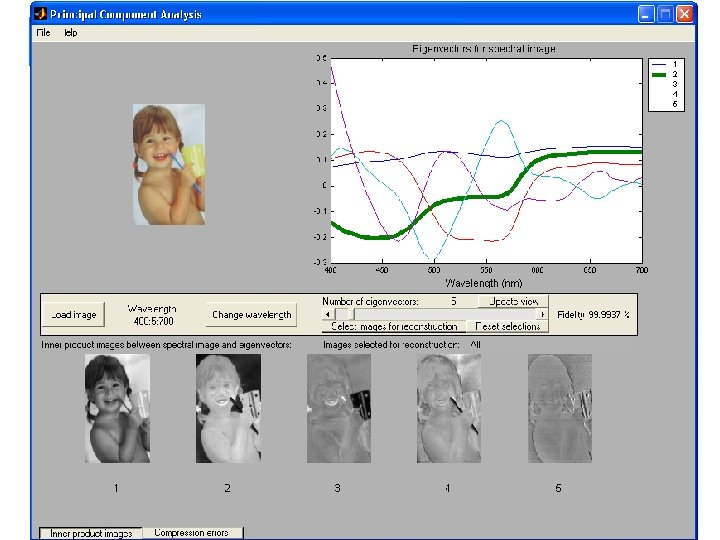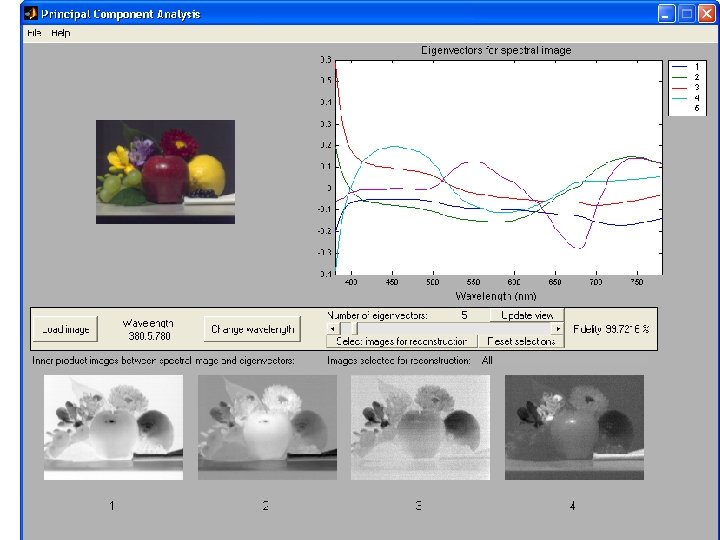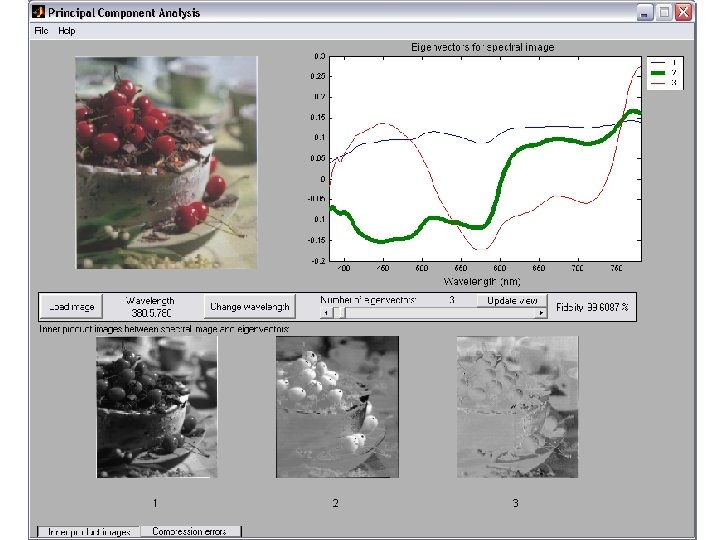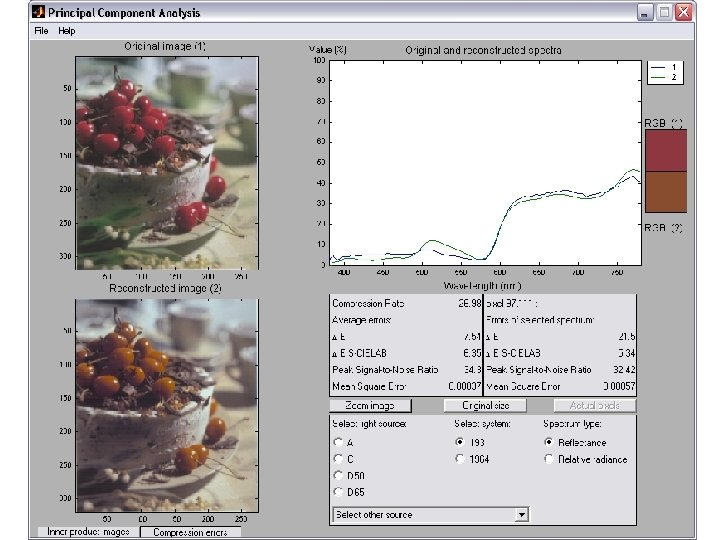An example of the PCA for the spectral image of printed productEigenvectors in case of the pure radiance data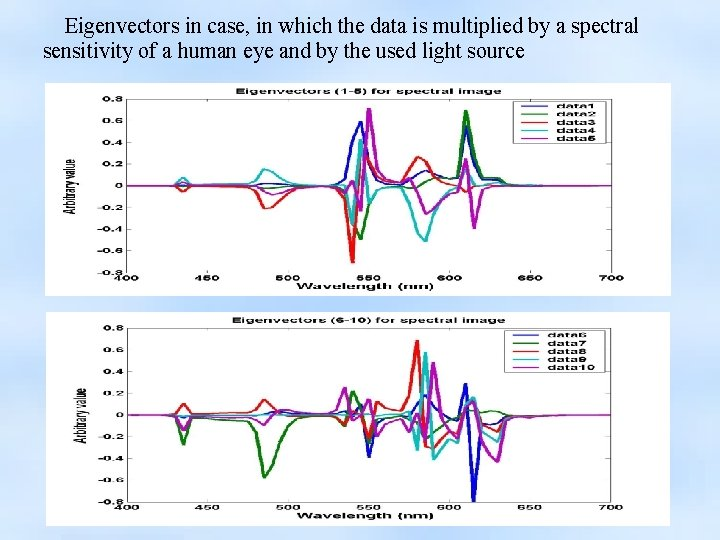Eigenvectors in case, in which the data is multiplied by a spectral sensitivity of a human eye and by the used light sourceInner-product images between the spectral images and eigenvectors Inner-product images between eigenvectors and data, which is multiplied by a spectral sensitivity of a human eye and the used light sourceAn example of the PCA for the spectral image measured from the PDA-displaySample spectral images of a PDA-display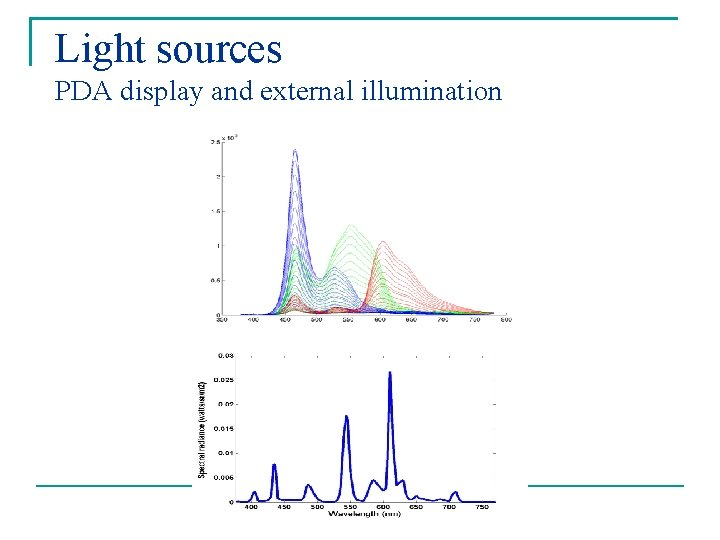Light sources PDA display and external illumination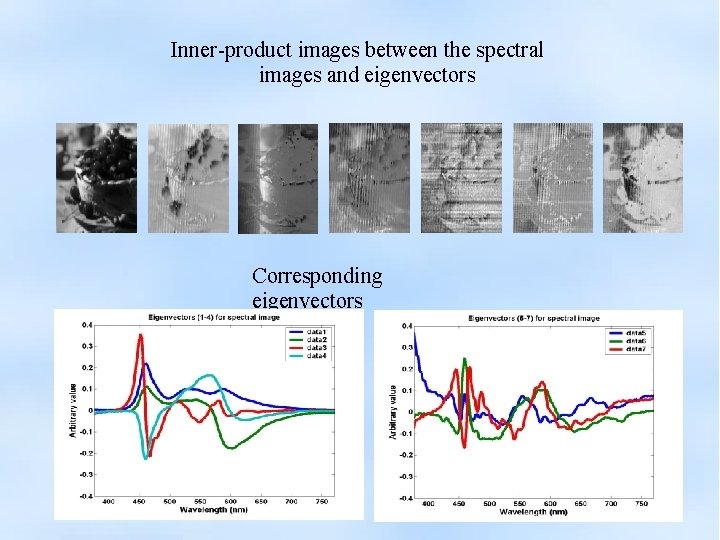Inner-product images between the spectral images and eigenvectors Corresponding eigenvectors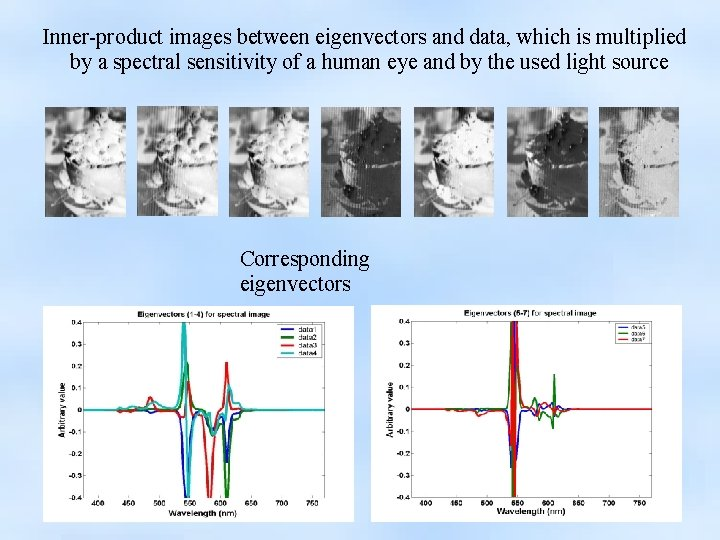Inner-product images between eigenvectors and data, which is multiplied by a spectral sensitivity of a human eye and by the used light source Corresponding eigenvectorsInner-product images between the spectral images and eigenvectors Corresponding eigenvectors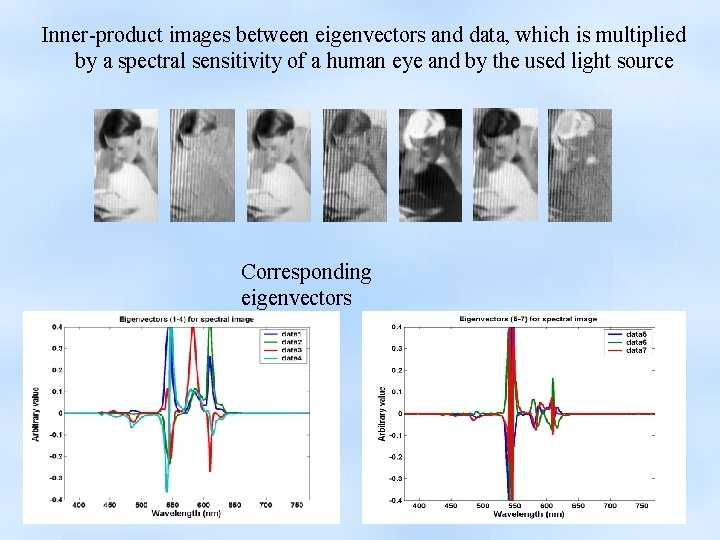Inner-product images between eigenvectors and data, which is multiplied by a spectral sensitivity of a human eye and by the used light source Corresponding eigenvectorsSummary PCA can be effectively used for spectral image compression PCA can be used for pattern recognition tasks Exercises on implementing the PCA